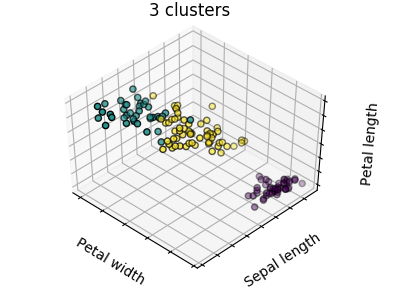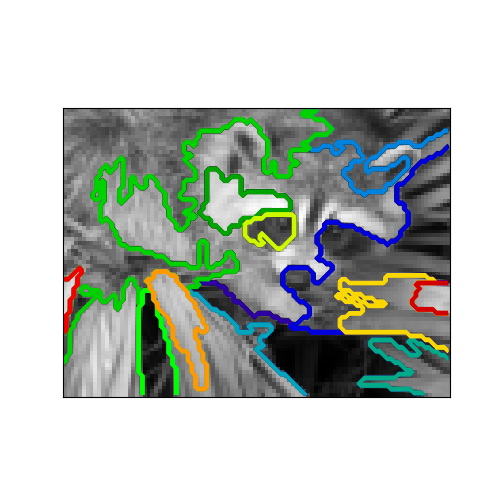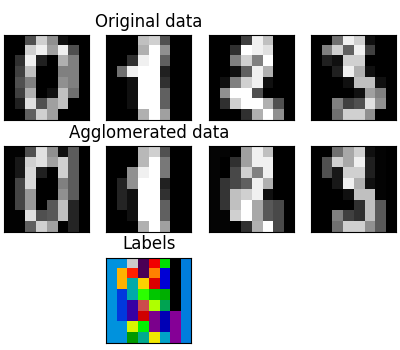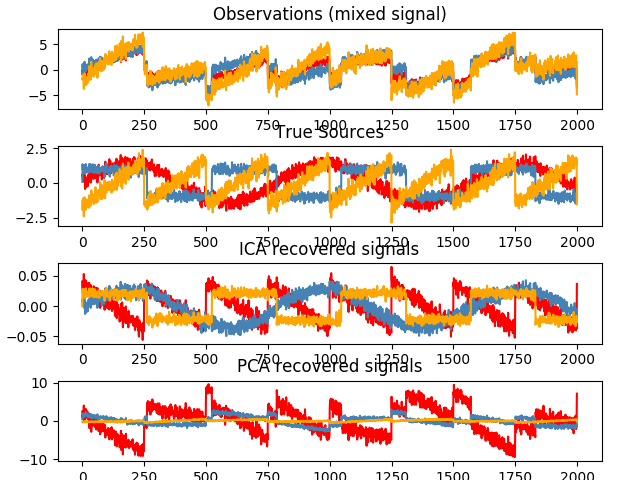# 无监督学习: 寻求数据表示

## 聚类: 对样本数据进行分组

• 对于 iris 数据集来说，我们知道所有样本有 3 种不同的类型，但是并不知道每一个样本是那种类型：此时我们可以尝试一个 clustering task（聚类任务） 聚类算法: 将样本进行分组，相似的样本被聚在一起，而不同组别之间的样本是有明显区别的，这样的分组方式就是 clusters（聚类）

### K-means 聚类算法>>> from sklearn import cluster, datasets
>>> X_iris = iris.data
>>> y_iris = iris.target

>>> k_means = cluster.KMeans(n_clusters=3)
>>> k_means.fit(X_iris)
KMeans(algorithm='auto', copy_x=True, init='k-means++', ...
>>> print(k_means.labels_[::10])
[1 1 1 1 1 0 0 0 0 0 2 2 2 2 2]
>>> print(y_iris[::10])
[0 0 0 0 0 1 1 1 1 1 2 2 2 2 2]



k_means 算法无法保证聚类结果完全绝对真实的反应实际情况。首先，选择正确合适的聚类数量不是一件容易的事情，第二，该算法对初始值的设置敏感，容易陷入局部最优。尽管 scikit-learn 采取了不同的方式来缓解以上问题，目前仍没有完美的解决方案。

• Don’t over-interpret clustering results（不要过分解读聚类结果）

Application example: vector quantization（应用示例:向量量化(vector quantization)）

• 一般来说聚类，特别是 K_means 聚类可以作为一种用少量样本来压缩信息的方式。这种方式就是 vector quantization 。例如，K_means 算法可以用于对一张图片进行色调分离:
>>> import scipy as sp
>>> try:
...    face = sp.face(gray=True)
... except AttributeError:
...    from scipy import misc
...    face = misc.face(gray=True)
>>> X = face.reshape((-1, 1)) # We need an (n_sample, n_feature) array
>>> k_means = cluster.KMeans(n_clusters=5, n_init=1)
>>> k_means.fit(X)
KMeans(algorithm='auto', copy_x=True, init='k-means++', ...
>>> values = k_means.cluster_centers_.squeeze()
>>> labels = k_means.labels_
>>> face_compressed = np.choose(labels, values)
>>> face_compressed.shape = face.shape


### 分层聚类算法: 谨慎使用

• Agglomerative（聚合） - 自底向上的方法: 初始阶段，每一个样本将自己作为单独的一个簇，聚类的簇以最小化距离的标准进行迭代聚合。当感兴趣的簇只有少量的样本时，该方法是很合适的。如果需要聚类的 簇数量很大，该方法比K_means算法的计算效率也更高。
• Divisive（分裂） - 自顶向下的方法: 初始阶段，所有的样本是一个簇，当一个簇下移时，它被迭代的进 行分裂。当估计聚类簇数量较大的数据时，该算法不仅效率低(由于样本始于一个簇，需要被递归的进行 分裂)，而且从统计学的角度来讲也是不合适的。

#### 连接约束聚类from scipy.ndimage.filters import gaussian_filter

import matplotlib.pyplot as plt

import skimage
from skimage.data import coins
from skimage.transform import rescale

from sklearn.feature_extraction.image import grid_to_graph
from sklearn.cluster import AgglomerativeClustering

# these were introduced in skimage-0.14
if LooseVersion(skimage.__version__) >= '0.14':
rescale_params = {'anti_aliasing': False, 'multichannel': False}
else:
rescale_params = {}

# #############################################################################
# Generate data
orig_coins = coins()

# Resize it to 20% of the original size to speed up the processing
# Applying a Gaussian filter for smoothing prior to down-scaling
# reduces aliasing artifacts.
smoothened_coins = gaussian_filter(orig_coins, sigma=2)


#### 特征聚集>>> digits = datasets.load_digits()
>>> images = digits.images
>>> X = np.reshape(images, (len(images), -1))
>>> connectivity = grid_to_graph(*images.shape)

>>> agglo = cluster.FeatureAgglomeration(connectivity=connectivity,
...                                      n_clusters=32)
>>> agglo.fit(X)
FeatureAgglomeration(affinity='euclidean', compute_full_tree='auto',...
>>> X_reduced = agglo.transform(X)

>>> X_approx = agglo.inverse_transform(X_reduced)
>>> images_approx = np.reshape(X_approx, images.shape)


transform and inverse_transform methods

• Some estimators expose a transform method, for instance to reduce the dimensionality of the dataset.

## 分解: 将一个信号转换成多个成份并且加载

• 如果 X 是多维数据，那么我们试图解决的问题是在不同的观察基础上对数据进行重写。我们希望学习得到载荷 L 和成分 C 使得 X = L C 。提取成分 C 有多种不同的方法。

### 主成份分析: PCA

>>> # Create a signal with only 2 useful dimensions
>>> x1 = np.random.normal(size=100)
>>> x2 = np.random.normal(size=100)
>>> x3 = x1 + x2
>>> X = np.c_[x1, x2, x3]

>>> from sklearn import decomposition
>>> pca = decomposition.PCA()
>>> pca.fit(X)
PCA(copy=True, iterated_power='auto', n_components=None, random_state=None,
svd_solver='auto', tol=0.0, whiten=False)
>>> print(pca.explained_variance_)
[  2.18565811e+00   1.19346747e+00   8.43026679e-32]

>>> # As we can see, only the 2 first components are useful
>>> pca.n_components = 2
>>> X_reduced = pca.fit_transform(X)
>>> X_reduced.shape
(100, 2)


### 独立成分分析: ICA>>> # Generate sample data
>>> import numpy as np
>>> from scipy import signal
>>> time = np.linspace(0, 10, 2000)
>>> s1 = np.sin(2 * time)  # Signal 1 : sinusoidal signal
>>> s2 = np.sign(np.sin(3 * time))  # Signal 2 : square signal
>>> s3 = signal.sawtooth(2 * np.pi * time)  # Signal 3: saw tooth signal
>>> S = np.c_[s1, s2, s3]
>>> S += 0.2 * np.random.normal(size=S.shape)  # Add noise
>>> S /= S.std(axis=0)  # Standardize data
>>> # Mix data
>>> A = np.array([[1, 1, 1], [0.5, 2, 1], [1.5, 1, 2]])  # Mixing matrix
>>> X = np.dot(S, A.T)  # Generate observations

>>> # Compute ICA
>>> ica = decomposition.FastICA()
>>> S_ = ica.fit_transform(X)  # Get the estimated sources
>>> A_ = ica.mixing_.T
>>> np.allclose(X,  np.dot(S_, A_) + ica.mean_)
True